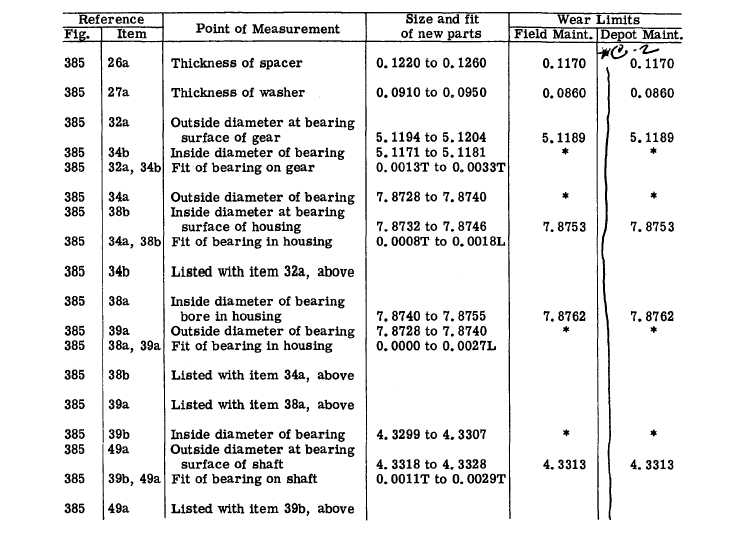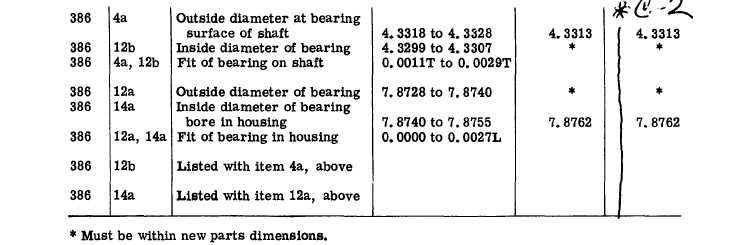R I G H T - O U T P U T   D R I V E   A S S E M B L Y   -   R E P A I R   A N D   R E B U I L D   S T A N D A R D S
Custom Search*C2 *C2 CHAP   6 R E P A I R     A N D     R E B U I L D     S T A N D A R DS P A R   2 4 8 - 2 4 9 2 4 9 .    R I G H T - O U T P U T    D R I V E    A S S E M B L Y    -    R E P A I R    A N D    R E B U I L D    S T A N D A R D S 2 2 8Integrated Publishing, Inc. - A (SDVOSB) Service Disabled Veteran Owned Small Business